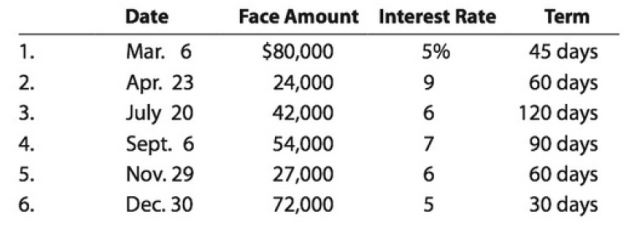Chapter 9, Problem 9.4APR

Chapter
Section
Textbook Problem

Details of notes receivable and related entriesFlush Mate Co. wholesales bathroom fixtures. During the current fiscal year, Flush Mate Co. received the following notes:Instructions 1. Determine for each note (a) the due date and (b) the amount of interest due at maturity, identifying each note by number. 2. Journalize the entry to record the dishonor of Note (3) on its due date. 3. Journalize the adjusting entry to record the accrued interest on Notes (5) and (6) on December 31. 4. Journalize the entries to record the receipt of the amounts due on Notes (5) and (6) in January.

(1)

To determine

Note receivable:

Note receivable refers to a written promise received by the creditor from the debtor in formal, for the amounts to be settled within a stipulated period of time. This written promise is issued by a debtor or borrower to the lender or creditor. Notes receivable is an asset of a business. Notes receivable often used for the credit periods of more than 60 days.

Due date:

Due date is the maturity date on note, on due date the borrower is supposed to repay the face value of the note along with interest.

Interest on note:

Interest on note is the amount charged on the principal value of note for the privilege of borrowing money. Interest is to be paid by the borrower and to be received by the lender.

To determine: (a) the due date and (b) the amount of interest due at maturity.

Explanation

Determine (a) the due date and (b) the amount of interest due at maturity.

 Due date Amount of interest due at maturity 1. April 20 $500 (1) 2. June 22$360 (2) 3. November 17 $840 (3) 4. December 5$945 (4) 5. January 28 $270 (5) 6. January 29$300 (6)

Table (1)

Working note:

For note 1:

Calculate the amount of interest due at maturity.

Total interest=[Face amount ×Annual interest rate×Time in terms of year]=[$80,000×5%100×45 days360 days]=$500 (1)

For note 2:

Calculate the amount of interest due at maturity.

Total interest=[Face amount ×Annual interest rate×Time in terms of year]=[$24,000×9%100×60 days360 days]=$360 (2)

For note 3:

Calculate the amount of interest due at maturity.

Total interest=[Face amount ×Annual interest rate×Time in terms of year]=[$42,000×6%100×120 days360 days]=$840 (3)

For note 4:

Calculate the amount of interest due at maturity

(2)

To determine

Dishonored note:

Note receivable refers to a written promise by the debtor for the amounts to be received within a stipulated period of time. Note is otherwise known as promissory note. If this promissory note is not settled by the debtor at its maturity date, then it became is known as dishonored note.

To journalize: The dishonor of Note (3) on its due date.

(3)

To determine

To journalize: The adjusting entry to record the accrued interest on Notes (5) and (6) on December 31.

(4)

To determine

To journalize: The entries to record the receipt of the amounts due on Notes (5) and (6) in January.

Still sussing out bartleby?

Check out a sample textbook solution.

See a sample solution

The Solution to Your Study Problems

Bartleby provides explanations to thousands of textbook problems written by our experts, many with advanced degrees!

Get Started

How can team conflict be reduced?

Foundations of Business (MindTap Course List)

What is employee fraud?

Accounting Information Systems

If a companys beta were to double, would its required return also double?

Fundamentals of Financial Management (MindTap Course List)

EXPECTED INTEREST RATE The real risk-free rate is 3%. Inflation is expected to be 3% this year, 4% next year, a...

Fundamentals of Financial Management, Concise Edition (with Thomson ONE - Business School Edition, 1 term (6 months) Printed Access Card) (MindTap Course List)